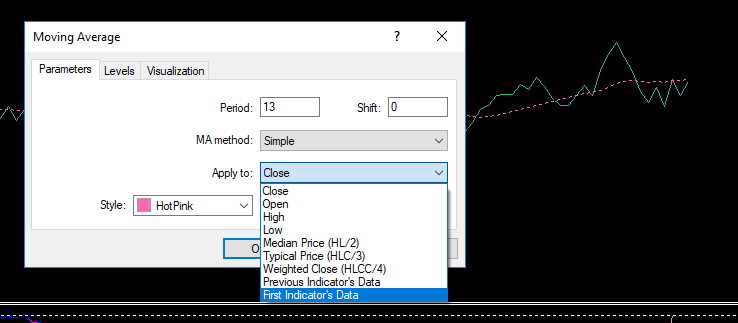# Another iMAOnArray problem636

Hi Guys,

I am trying to extract MA values (with iMAOnArray) when I attach a 13 SMA to the OBV indicator by drag and drop using the First Indicator option:My code is below and the param2 values are all zero. I have read many of the other posts about iMAOnArray and I think I have used it correctly here but would appreciate comments from anybody otherwise.

```double param1, param2;

void OnStart()
{
//fill OBV array first
for(int i=0;i<50;i++)
{
param1[i] = iOBV(NULL,0,PRICE_CLOSE,i);
//Print(i,"   ",param1[i]);
}
ArraySetAsSeries(param1,True);
for(i=0;i<50;i++)
{
param2 = iMAOnArray(param1,0,13,0,MODE_SMA,i);
Print(i,"  ",param2);
}
}```20228

You would know what the problem is, if you had used strict.

Always use strict. Fixing the warnings will save you hours of debugging.636

William Roeder:
You would know what the problem is, if you had used strict.

Always use strict. Fixing the warnings will save you hours of debugging.

I normally do, so thanks for the reminder.

I'm now getting values with the repaired code but regardless of whether I use ArraySetAsSeries or not the values appear in reverse order which is correct for standard use of iMAOnArray which calculates from left to right.

I understood that ArraySetAsSeries reverses the index order from right to left. Please can you suggest where I am going wrong. Thank you.

```#property strict

double param1, param2;

void OnStart()
{
//fill OBV array first
for(int i=0;i<50;i++)
{
param1[i] = iOBV(NULL,0,PRICE_CLOSE,i);
//Print(i,"   ",param1[i]);
}
ArraySetAsSeries(param1,True);
for(int i=0;i<50;i++)
{
param2 = iMAOnArray(param1,0,13,0,MODE_SMA,i);
Print(i,"  ",DoubleToStr(param2,0));
}
}```Moderator
20477

sd59:

I normally do, so thanks for the reminder.

I'm now getting values with the repaired code but regardless of whether I use ArraySetAsSeries or not the values appear in reverse order which is correct for standard use of iMAOnArray which calculates from left to right.

I understood that ArraySetAsSeries reverses the index order from right to left. Please can you suggest where I am going wrong. Thank you.

I don't believe that the documentation is very clear.

You should set the array as series before filling it.

```void OnStart()
{
ArraySetAsSeries(param1,True);
//fill OBV array first
for(int i=0;i<50;i++)
{
param1[i] = iOBV(NULL,0,PRICE_CLOSE,i);
//Print(i,"   ",param1[i]);
}
//ArraySetAsSeries(param1,True);
for(int i=0;i<50;i++)
{
param2 = iMAOnArray(param1,0,13,0,MODE_SMA,i);
Print(i,"  ",DoubleToStr(param2,0));
}
//---

}```636

Keith Watford:

I don't believe that the documentation is very clear.

You should set the array as series before filling it.

Brilliant! Thank you.636

Just to continue this post on the same subject I'm having problems getting the correct values from iMAOnArray when I add (by drag and drop) a 50 SMA to a custom indicator I have.

I can confirm that the iCustom call does return the correct values of the indicator and I do get values returned by iMAOnArray except not matching the ones shown in the Data window.

I wanted to ask if my code is correct and any other suggestions please?

```#property strict

double average;

void OnStart()
{
ArrayResize(sell,Bars-1,100);
ArrayResize(total,Bars-1,100);
ArraySetAsSeries(total,True);

for(int i=0;i<Bars-1;i++)
{
sell[i] = iCustom(NULL,0,"SD VSA",1,i);
Print(total[i]);
}

for(int i=0;i<Bars-1;i++){
average = iMAOnArray(total,0,50,0,MODE_SMA,i);
Print(average);}

}```20228

Answered in #3 then you broke it.636

William Roeder:
Answered in #3 then you broke it.
Not very helpful - please don't respond unless you have a sensible contribution.